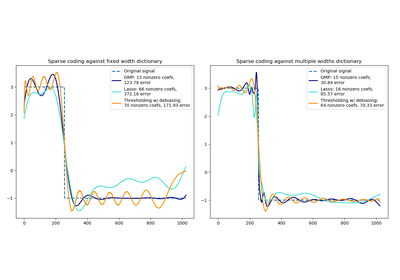# `sklearn.decomposition`.SparseCoder¶

class `sklearn.decomposition.``SparseCoder`(dictionary, transform_algorithm=’omp’, transform_n_nonzero_coefs=None, transform_alpha=None, split_sign=False, n_jobs=1)[source]

Sparse coding

Finds a sparse representation of data against a fixed, precomputed dictionary.

Each row of the result is the solution to a sparse coding problem. The goal is to find a sparse array code such that:

```X ~= code * dictionary
```

Read more in the User Guide.

Parameters: dictionary : array, [n_components, n_features] The dictionary atoms used for sparse coding. Lines are assumed to be normalized to unit norm. transform_algorithm : {‘lasso_lars’, ‘lasso_cd’, ‘lars’, ‘omp’, ‘threshold’} Algorithm used to transform the data: lars: uses the least angle regression method (linear_model.lars_path) lasso_lars: uses Lars to compute the Lasso solution lasso_cd: uses the coordinate descent method to compute the Lasso solution (linear_model.Lasso). lasso_lars will be faster if the estimated components are sparse. omp: uses orthogonal matching pursuit to estimate the sparse solution threshold: squashes to zero all coefficients less than alpha from the projection `dictionary * X'` transform_n_nonzero_coefs : int, `0.1 * n_features` by default Number of nonzero coefficients to target in each column of the solution. This is only used by algorithm=’lars’ and algorithm=’omp’ and is overridden by alpha in the omp case. transform_alpha : float, 1. by default If algorithm=’lasso_lars’ or algorithm=’lasso_cd’, alpha is the penalty applied to the L1 norm. If algorithm=’threshold’, alpha is the absolute value of the threshold below which coefficients will be squashed to zero. If algorithm=’omp’, alpha is the tolerance parameter: the value of the reconstruction error targeted. In this case, it overrides n_nonzero_coefs. split_sign : bool, False by default Whether to split the sparse feature vector into the concatenation of its negative part and its positive part. This can improve the performance of downstream classifiers. n_jobs : int, number of parallel jobs to run components_ : array, [n_components, n_features] The unchanged dictionary atoms

Methods

 `fit`(X[, y]) Do nothing and return the estimator unchanged `fit_transform`(X[, y]) Fit to data, then transform it. `get_params`([deep]) Get parameters for this estimator. `set_params`(**params) Set the parameters of this estimator. `transform`(X) Encode the data as a sparse combination of the dictionary atoms.
`__init__`(dictionary, transform_algorithm=’omp’, transform_n_nonzero_coefs=None, transform_alpha=None, split_sign=False, n_jobs=1)[source]
`fit`(X, y=None)[source]

Do nothing and return the estimator unchanged

This method is just there to implement the usual API and hence work in pipelines.

Parameters: X : Ignored. y : Ignored. self : object Returns the object itself
`fit_transform`(X, y=None, **fit_params)[source]

Fit to data, then transform it.

Fits transformer to X and y with optional parameters fit_params and returns a transformed version of X.

Parameters: X : numpy array of shape [n_samples, n_features] Training set. y : numpy array of shape [n_samples] Target values. X_new : numpy array of shape [n_samples, n_features_new] Transformed array.
`get_params`(deep=True)[source]

Get parameters for this estimator.

Parameters: deep : boolean, optional If True, will return the parameters for this estimator and contained subobjects that are estimators. params : mapping of string to any Parameter names mapped to their values.
`set_params`(**params)[source]

Set the parameters of this estimator.

The method works on simple estimators as well as on nested objects (such as pipelines). The latter have parameters of the form `<component>__<parameter>` so that it’s possible to update each component of a nested object.

Returns: self :
`transform`(X)[source]

Encode the data as a sparse combination of the dictionary atoms.

Coding method is determined by the object parameter transform_algorithm.

Parameters: X : array of shape (n_samples, n_features) Test data to be transformed, must have the same number of features as the data used to train the model. X_new : array, shape (n_samples, n_components) Transformed data

## Examples using `sklearn.decomposition.SparseCoder`¶Sparse coding with a precomputed dictionary